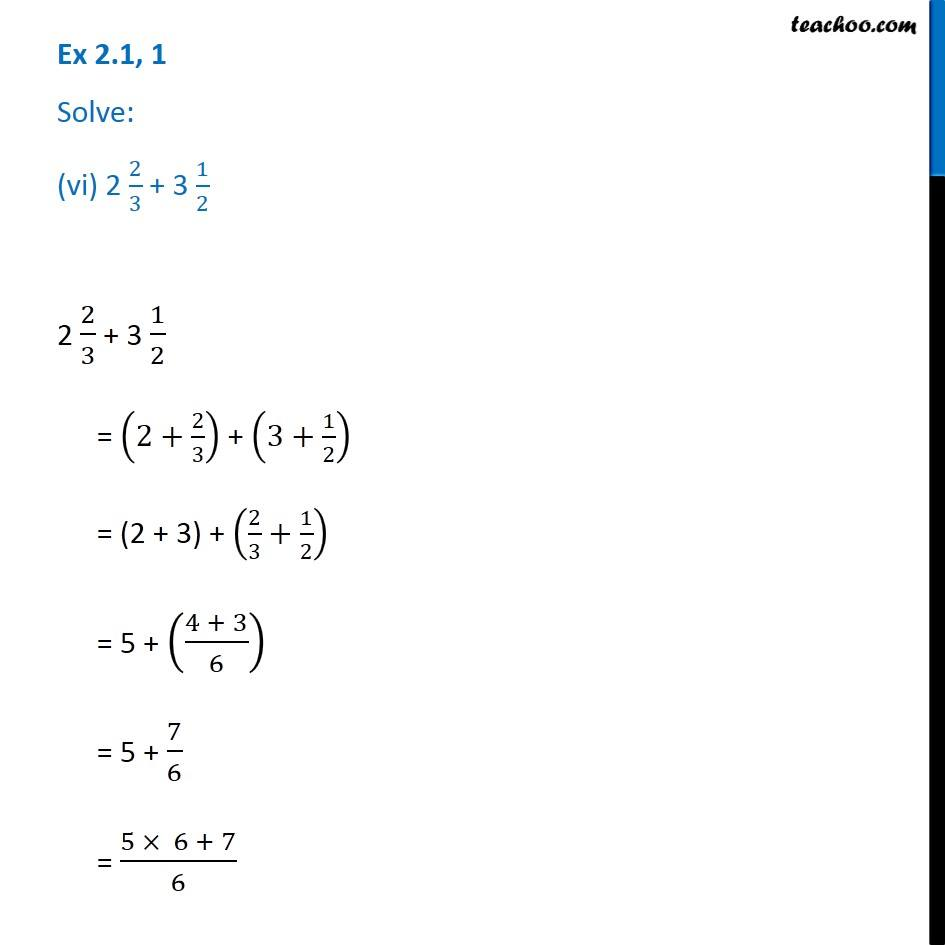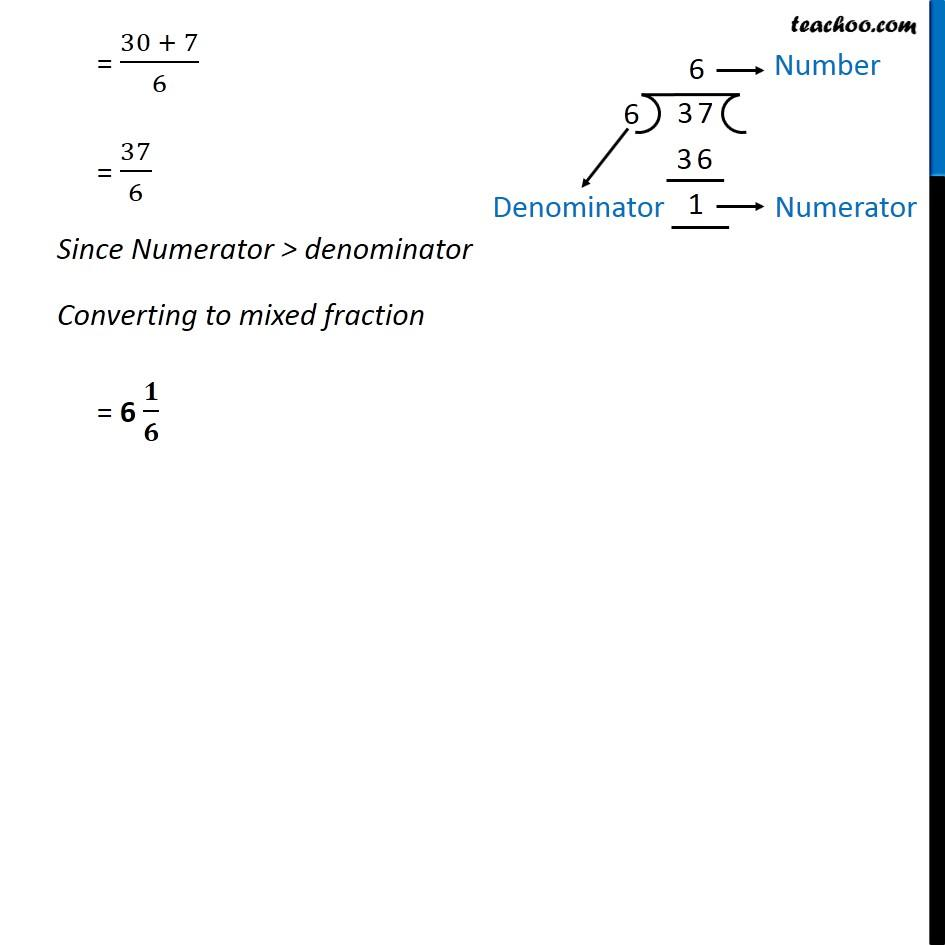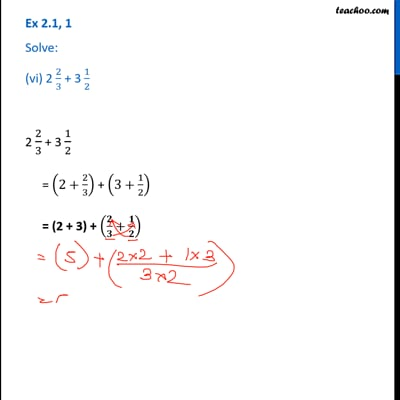Ex 2.1

Chapter 2 Class 7 Fractions and Decimals
Serial order wiseThis video is only available for Teachoo black users

Get live Maths 1-on-1 Classs - Class 6 to 12

### Transcript

Ex 2.1, 1 Solve: (vi) 2 2/3 + 3 1/2 2 2/3 + 3 1/2 = (2+2/3) + (3+1/2) = (2 + 3) + (2/3+1/2) = 5 + ((4 + 3)/6) = 5 + 7/6 = (5 × 6 + 7)/6 = (30 + 7)/6 = 37/6 Since Numerator > denominator Converting to mixed fraction = 6 𝟏/𝟔